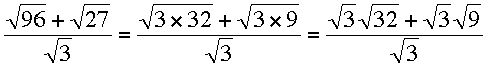SEARCH HOMEMath Central Quandaries & QueriesQuestion from linda, a student: radical 96 plus radical 27 divide by radical 3Hi Linda,

What you need to see here is that 96 and 27 are both divisible by 3, 96 = 3 × 32 and 27 = 3 × 9. hence you can writeContinue to simplify.

PennyMath Central is supported by the University of Regina and The Pacific Institute for the Mathematical Sciences.